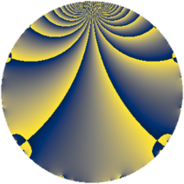Properties

 Label 847.2.aLevel $847$ Weight $2$ Character orbit 847.a Rep. character $\chi_{847}(1,\cdot)$ Character field $\Q$ Dimension $55$ Newform subspaces $16$ Sturm bound $176$ Trace bound $3$

Related objects

Defining parameters

 Level: $$N$$ $$=$$ $$847 = 7 \cdot 11^{2}$$ Weight: $$k$$ $$=$$ $$2$$ Character orbit: $$[\chi]$$ $$=$$ 847.a (trivial) Character field: $$\Q$$ Newform subspaces: $$16$$ Sturm bound: $$176$$ Trace bound: $$3$$ Distinguishing $$T_p$$: $$2$$, $$3$$

Dimensions

The following table gives the dimensions of various subspaces of $$M_{2}(\Gamma_0(847))$$.

Total New Old
Modular forms 100 55 45
Cusp forms 77 55 22
Eisenstein series 23 0 23

The following table gives the dimensions of the cuspidal new subspaces with specified eigenvalues for the Atkin-Lehner operators and the Fricke involution.

$$7$$$$11$$FrickeDim.
$$+$$$$+$$$$+$$$$13$$
$$+$$$$-$$$$-$$$$15$$
$$-$$$$+$$$$-$$$$17$$
$$-$$$$-$$$$+$$$$10$$
Plus space$$+$$$$23$$
Minus space$$-$$$$32$$

Trace form

 $$55 q - q^{2} - 4 q^{3} + 59 q^{4} + 2 q^{5} + 8 q^{6} - q^{7} + 3 q^{8} + 47 q^{9} + O(q^{10})$$ $$55 q - q^{2} - 4 q^{3} + 59 q^{4} + 2 q^{5} + 8 q^{6} - q^{7} + 3 q^{8} + 47 q^{9} + 2 q^{10} - 16 q^{12} + 2 q^{13} + q^{14} - 8 q^{15} + 55 q^{16} + 2 q^{17} + 19 q^{18} + 6 q^{20} - 4 q^{21} + 4 q^{23} + 16 q^{24} + 57 q^{25} - 18 q^{26} - 16 q^{27} - 7 q^{28} - 6 q^{29} - 16 q^{30} - 16 q^{31} - 5 q^{32} + 6 q^{34} - 2 q^{35} + 43 q^{36} + 2 q^{37} - 24 q^{38} - 8 q^{39} - 6 q^{40} + 10 q^{41} + 12 q^{42} - 28 q^{43} - 2 q^{45} - 16 q^{46} - 20 q^{47} - 8 q^{48} + 55 q^{49} + q^{50} - 4 q^{51} - 18 q^{52} - 10 q^{53} + 24 q^{54} - 3 q^{56} - 4 q^{57} - 30 q^{58} - 20 q^{60} + 22 q^{61} - 20 q^{62} + 3 q^{63} + 83 q^{64} + 20 q^{65} - 36 q^{67} + 2 q^{68} - 16 q^{69} - 14 q^{70} + 23 q^{72} + 2 q^{73} + 26 q^{74} - 32 q^{75} - 20 q^{76} - 28 q^{78} - 4 q^{79} - 54 q^{80} + 15 q^{81} - 14 q^{82} - 28 q^{83} + 24 q^{85} - 44 q^{86} + 60 q^{87} - 2 q^{89} - 38 q^{90} + 2 q^{91} - 36 q^{92} - 32 q^{93} + 20 q^{94} - 4 q^{95} - 40 q^{96} - 10 q^{97} - q^{98} + O(q^{100})$$

Decomposition of $$S_{2}^{\mathrm{new}}(\Gamma_0(847))$$ into newform subspaces

Label Dim. $$A$$ Field CM Traces A-L signs $q$-expansion
$a_{2}$ $a_{3}$ $a_{5}$ $a_{7}$ 7 11
847.2.a.a $1$ $6.763$ $$\Q$$ None $$-1$$ $$2$$ $$-2$$ $$1$$ $-$ $-$ $$q-q^{2}+2q^{3}-q^{4}-2q^{5}-2q^{6}+q^{7}+\cdots$$
847.2.a.b $1$ $6.763$ $$\Q$$ None $$0$$ $$-3$$ $$-1$$ $$1$$ $-$ $-$ $$q-3q^{3}-2q^{4}-q^{5}+q^{7}+6q^{9}+6q^{12}+\cdots$$
847.2.a.c $1$ $6.763$ $$\Q$$ None $$0$$ $$1$$ $$3$$ $$-1$$ $+$ $-$ $$q+q^{3}-2q^{4}+3q^{5}-q^{7}-2q^{9}-2q^{12}+\cdots$$
847.2.a.d $2$ $6.763$ $$\Q(\sqrt{5})$$ None $$-3$$ $$-1$$ $$2$$ $$2$$ $-$ $-$ $$q+(-1-\beta )q^{2}+(-1+\beta )q^{3}+3\beta q^{4}+\cdots$$
847.2.a.e $2$ $6.763$ $$\Q(\sqrt{13})$$ None $$-1$$ $$-1$$ $$0$$ $$2$$ $-$ $-$ $$q-\beta q^{2}-\beta q^{3}+(1+\beta )q^{4}+(-1+2\beta )q^{5}+\cdots$$
847.2.a.f $2$ $6.763$ $$\Q(\sqrt{5})$$ None $$0$$ $$2$$ $$-4$$ $$-2$$ $+$ $-$ $$q-\beta q^{2}+(1-\beta )q^{3}+3q^{4}-2q^{5}+(5+\cdots)q^{6}+\cdots$$
847.2.a.g $2$ $6.763$ $$\Q(\sqrt{13})$$ None $$1$$ $$-1$$ $$0$$ $$-2$$ $+$ $-$ $$q+\beta q^{2}-\beta q^{3}+(1+\beta )q^{4}+(-1+2\beta )q^{5}+\cdots$$
847.2.a.h $2$ $6.763$ $$\Q(\sqrt{5})$$ None $$3$$ $$-1$$ $$2$$ $$-2$$ $+$ $-$ $$q+(1+\beta )q^{2}+(-1+\beta )q^{3}+3\beta q^{4}+\cdots$$
847.2.a.i $3$ $6.763$ 3.3.568.1 None $$-2$$ $$-1$$ $$1$$ $$-3$$ $+$ $+$ $$q+(-1+\beta _{1})q^{2}-\beta _{1}q^{3}+(3+\beta _{2})q^{4}+\cdots$$
847.2.a.j $3$ $6.763$ 3.3.568.1 None $$2$$ $$-1$$ $$1$$ $$3$$ $-$ $+$ $$q+(1-\beta _{1})q^{2}-\beta _{1}q^{3}+(3+\beta _{2})q^{4}+\cdots$$
847.2.a.k $4$ $6.763$ 4.4.2525.1 None $$-2$$ $$-2$$ $$-6$$ $$4$$ $-$ $-$ $$q-\beta _{1}q^{2}+(-1-\beta _{2})q^{3}+(1+\beta _{1}+\beta _{2}+\cdots)q^{4}+\cdots$$
847.2.a.l $4$ $6.763$ 4.4.2525.1 None $$2$$ $$-2$$ $$-6$$ $$-4$$ $+$ $+$ $$q+\beta _{1}q^{2}+(-1-\beta _{2})q^{3}+(1+\beta _{1}+\beta _{2}+\cdots)q^{4}+\cdots$$
847.2.a.m $6$ $6.763$ 6.6.7674048.1 None $$-4$$ $$-2$$ $$-4$$ $$-6$$ $+$ $+$ $$q+(-1+\beta _{1})q^{2}+\beta _{5}q^{3}+(1-\beta _{1}+\beta _{2}+\cdots)q^{4}+\cdots$$
847.2.a.n $6$ $6.763$ 6.6.7674048.1 None $$4$$ $$-2$$ $$-4$$ $$6$$ $-$ $+$ $$q+(1-\beta _{1})q^{2}+\beta _{5}q^{3}+(1-\beta _{1}+\beta _{2}+\cdots)q^{4}+\cdots$$
847.2.a.o $8$ $6.763$ $$\mathbb{Q}[x]/(x^{8} - \cdots)$$ None $$-1$$ $$4$$ $$10$$ $$-8$$ $+$ $-$ $$q-\beta _{1}q^{2}+(1-\beta _{4}-\beta _{7})q^{3}+(1+\beta _{5}+\cdots)q^{4}+\cdots$$
847.2.a.p $8$ $6.763$ $$\mathbb{Q}[x]/(x^{8} - \cdots)$$ None $$1$$ $$4$$ $$10$$ $$8$$ $-$ $+$ $$q+\beta _{1}q^{2}+(1-\beta _{4}-\beta _{7})q^{3}+(1+\beta _{5}+\cdots)q^{4}+\cdots$$

Decomposition of $$S_{2}^{\mathrm{old}}(\Gamma_0(847))$$ into lower level spaces

$$S_{2}^{\mathrm{old}}(\Gamma_0(847)) \cong$$ $$S_{2}^{\mathrm{new}}(\Gamma_0(11))$$$$^{\oplus 4}$$$$\oplus$$$$S_{2}^{\mathrm{new}}(\Gamma_0(77))$$$$^{\oplus 2}$$$$\oplus$$$$S_{2}^{\mathrm{new}}(\Gamma_0(121))$$$$^{\oplus 2}$$